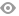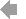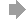﻿ Page 7 - Gateways_Fall2021
Basic HTML VersionView Full Version
Page 7 - Gateways_Fall2021
P. 7

Arrival at Brookfield Zoo: JP’s birth date is May 16, 2017, JP’s birth date is May 16, 2017,   Fun facts:
u
o
g
s
a K a
t
e i
u
n
t
n
n t
d h
r
s, A
s t
d c
i
r
e
o
c
n
a
a
n
lf b
d H
e s
i
h
e
a
o p
r
a and he is the second calf born to parents, Augusta K and Hiari.  .      Okapi are not related to zebras or antelopes; their closest
e
a
r
s
e
b
t
n
l
e
s o
s
o
r a
k
e n
t r
o
p
i a
a
r
O
d t
e
o z
t
l
e
t
a
l

h
p
t
;
e
o
e
r c
s
i
d o
m
t
n
m
s b
a
i
h
o
e
d
n a r
r
n
t
i
n
a
g o
i
n
s w
a
e p
f h
a
i
The pairing of his parents was based on a recommendation     relative is the giraffe. .
a
c
s
T
s p
e
o
r
e
e
s
v
e
ti
i
th
g

i
r
aff
e
a
el
r
e
a
o
d A
’ O
s a
s
k
s
o
c
r
n
ie
s
c
m t
f
o
u
from the Association of Zoos and Aquariums’ Okapi Species       The okapi’s stripes help them blend in with their forest
s
m
o
i
n o
i
o
e
p
i
q
e A
p
r
t
i S
f Z
a
a
u
h
t
i
e
h
e
h
p
e
n
’
s s
T
it
i
d i
p t
k
e
m b
r
t
h t
s h
n w
o
a
h
i
l
r f
r
l
s
e o
e
p
e
a
P)
t
a
i
en
v
n
c
t
p
em
o
g
o
u
a
ma
n
p
a
o
,
l
er
t
i
o
p
e
v
P
S
n
a
l
al
i
u
r
Survival Plan® (SSP), a cooperative population management     surroundings to escape predators.  .
S
(S
v
a
p
sc
t
s
e
o
t
a
o
s
r
d

e
p
e
r
r
nd
s
i
u
o
u
r
n
g
n
i
o
n
m w
n
a
o
t
i
a
i
g

o
h t
and conservation program with the goal of maintaining
h
e
a
d c
n p
a
f m
n
it
l o
a
s
o
a
r
g
g
e
n
t
r
i
v
r
u
a
d p
q
e
s h
’
i
d
t
n
iq
a
n
u
T
t
e
t
r
t
e
s u
s i
r
n o
k
r
p
a
e
n a
n o
e s
h
p
i
i
r
genetic diversity and demographic stability of a species in
g e n e t i c d i v e r s it y a n d d e m o g r a p h i c s t a b i l it y o f a s p e c ie s i n      The striped pattern on an okapi’s hindquarters is unique
h
l
t
l
e
i
t
e
p a c
t a
lf
a
s
i
a
a
s b
y h
i
o t
p
ie
e
h
c
t
r
v
t
m
ie
n
l
a
n
e s
s m
e t
. S
ag
g
e
r
d
t
e
c
n
.

-
e
s
s
n
a
managed-care settings.                             to that animal. Scientists believe the stripes may help a calf
a
m
t
i
e
l
i
z
o
recognize its mother as it follows behind her.  .
e it
h
r
l
i
e
c
g
s it f
d h
o
e
r
n
e
r a
n
o
o
ws b
s m
t
h
His name: JP was named after the immediate past chair  JP was named after the immediate past chair     Scent glands on their hooves secrete chemicals that mark
t g
e
i
n
m
r h
i
c
v
s o
a
c
t
r
o
c
S
k
o
e
e c
n t
e
h
e
a
d
l
h
s s
s t
e
l
n
t m
a
e
a
h
r
o f   t h e C Z S B o a r d o f T r u s t e e s, J o h n P . G r u b e .
of the CZS Board of Trustees, John P. Grube.
s h
r
h t
o
h
h
e
a
a
e
o
h
r
t
u
their path through the forest. Their large ears help them hear
e
h
s
g
a
h t
r p
e f
t
m h
. T
g
a
t
e
r l
e
h
i
r
e e
e
r
l
i
p t
r
JP’s personality: He is sometimes shy around staff and He is sometimes shy around staff and   t the low-frequency sounds they use to communicate with
h
s t
e t
y u
s
e
r
f
y s
t
i
-
a
c
e
it
n
h
e
c
e w
u
q
n
o
u
m
e l
n
h
o c
w
d
u
o
o
m
i
o w
s a l
e
n
e t
m
a
g t
o
m u
p t
m
e
u
r
e
e
h
. W
k
s
w h
a
a
n
t takes a long time to warm up to new humans. When he     each other.
n h
o n
h
o
h
e
e
c
t
a
r.
meets a new okapi, he makes a lot of low “chuffing” noises.
m e e t s a n e w o k a p i , h e m a k e s a l o t o f l o w “ c h uffi n g ” n o i s e s .      An okapi calf spends the first two months of its life alone
n
p
s t
k
o
e fi
e
o
t
h
lf s
n o
w
s o
n
i c
h
a
l
p
f
e a
d
e
s
s l
r
i
o m
A
f it
t t
n
a
O k a p i a t B r o o k fi e ld Z o o o f t e n h a n g o u t t o g e t h e r a n d s p e n d    h i d d e n i n v e g e t a t i o n . I t s m o m r e t u r n s o n l y b r ie fl y t o n u r s e it .
Okapi at Brookfield Zoo often hang out together and spend
hidden in vegetation. Its mom returns only briefly to nurse it.
. J
t
m
s v
m
g e
i
a
d o
y f
o
o
n
time grooming each other. JP is very fond of female okapi  i    This keeps the calf safe from predators.  .
P i
f f
e g
l
m
r
i
k
r
e
p
a
n
e
h o
h
e
c
t
r
o
e o
a
e c
m p
e
p
o
t
e
a
o
e f
a
r
h
lf s
i
s t
s k
f
d
s
r
T
r
a
h
e
M a k e m b a . H e i s n ’ t a p i c k y e a t e r a n d h i s f a v o r it e f o o d i s m a p l e
Makemba. He isn’t a picky eater and his favorite food is maple
r
0 d
o
p f
o
o
e b
n
a
r 3
o
n
h
’
f
lv
e
t p
r t
t
a
e
o 6
y a
s a
y
r
e
o
0 t
C
s d
y b
r
r
b
ws
l
o
n
e
e (l
u
e
v
d m
a
)
s
r
a and mulberry browse (leaves).                     Calves don’t poop for 30 to 60 days after they are born.  .
.
e
o
e d
o
r
w
e f
n
a
d
r
t
e
s t
t w
a
h
r
ld b
a
f
a
u
r
o
m p
p
h
T This keeps them safe from predators that would be drawn
h
s t
e
e
k

i
s
e
m s
Conservation threats: Habitat loss and destruction caused Habitat loss and destruction caused   by the smell. .
t
e
y
b
sm

ll
h
e
b y l o g g i n g , f a r m i n g , m i n i n g , h u m a n s e t t l e m e n t , a n d w a r .
by logging, farming, mining, human settlement, and war.
O k a p i a r e i l l e g a l l y h u n t e d f o r t h e i r s k i n a n d m e a t .
Okapi are illegally hunted for their skin and meat.
What CZS is doing:  In 1959, an okapi was born at In 1959, an okapi was born at
o
ld
t
p
e
B
Brookfield Zoo —the first okapi born in a North American
o
h
i b
k
r
n i
c
o
r
i
r
s
a
n
e
e fi
o
k
n a N
a
fi
o

h A
m
t

—
r
Z
t o
o
r
i
o
ld Z
k
zoo.  Brookfield Zoo has been a leader in developing
o
e
e
o
z
n
g
a
o
n d
d
r
n a l
p
o
e
v
r i
a
o
e
.  B
e
e
s b
l
o h
fi
e
r o
n i
o
P i
c
. J
a
t
d
p
e a
d r
u
e
s t
n z
o
i
a
r
e
a
o
r
o
a
n
d
k
r
s
i c
h
s f
o
d
t
n
s standards for okapi care and reproduction in zoos. JP is the
p
s p
p
a
a
t
o
t o
8
h o
e
f a n
o
o
ld Z
S i
t B
k o
28th okapi born at Brookfield Zoo. CZS is part of a network of
o
fi
r
k
r
r
o
r
o
i b
f
k
e
. C
t
2
w
Z
n a
o
o
z zoos around the world that support the Okapi Conservation
n
s
h
k
u
o
o
w
u
t s
a
o
r
n
h
o
d t
p
n

o
i C
e
i
r
p
p
t t
e O
e
h
s a
r
a
r
ld t
t
v
a
i
o
r
ld
l
e
c
d
i W
v
e
r
h
k
e O
n
s
p
t a
a
e

t
f
i
e R
P
je
Project and the Okapi Wildlife Reserve. .
SHARING the CARE
What you can do: Help us celebrate World Okapi Help us celebrate World Okapi
D a y o n O c t o b e r 1 8 . B r i n g i n y o u r o ld c e l l p h o n e s a n d    V i s it
Day on October 18. Bring in your old cell phones and
d
o
p
t
b
a
p
e
l
t
f d
o
t o
e l
i
s
n
s o
f a
l
z
e
e
a
o
m
r

o
c
v
s
e m
r
b
t
i
a
h
g
e
t tablets for recycling. These devices are made using                   f for a complete list of dozens of adoptable
a
n
d
s
i
e
e
l
n
i
g. T
o
r r
e
l
c
y
e
e d
e u
s f
c
s a
c
m
a
s
a
l
e

a
k
e
p
nim
pt
n
o
i
a
g
d
a
ck
o
nim
l
.
a
A
s
d
er
l
n
ta
i
t
M
mi
k
i

n
n
d
m
c
t
Na
al
e
l
e
o
al
n

o
c
s
i
ha
o
i
a a mineral called coltan that is mined in Maiko National  l            a animals. Animal adoption packages make
t
na
ai
great gifts and start at \$35. They include
P a r k a n d V i r u n g a N a t i o n a l P a r k , c r it i c a l o k a p i h a b it a t s .    g r e a t g i f t s a n d s t a r t a t \$ 3 5 . T h e y   i n c l u d e
Park and Virunga National Park, critical okapi habitats.

p
t
t
r
i
s
e
n c
a personalized adoption certificate, a 5”x7”
n
a
r
d
o
i
e
o
z
d a
”
, a 5
t
e
7
”
x
o
e
l
c
p
fi
a
i
a
, a s
h
o
t
c
c color photo of the animal, a species fact
p
o o
c
r p
s f
l
e
a
a
t
ie
e a
n
l
m
o
o
i
h
f t
d a p

f
, a
l
a
t
a
h
e
u
o
m
r
m
e
a
e
t
. S
e
o
c
e
d

r
e
n
sheet, and a program decal. Some featured
r
s
g
d
s
so
p
e
c
a animal packages also include a plush toy.  .
n
l
h
l
i
u
l
p
a
n
l
o
a
y
s
ud
ag
m
i
e
a
ac
t
k
a
i

, e
t
it
m
m
e

s
o
a
o
r
e
n
n
o
i
f
r
it o
s
, v
r
r w
o
e
m
i
b
i
u
e
o
y your question to SharetheCare@CZS.org,  ,
o
s
n t
o
r q
i
u
u
t
or call (708) 688-8341.
o r   c a l l   ( 7 0 8 ) 6 8 8 - 8 3 4 1 .
BROOKFIELD ZOO | FALL 2021  7 7 72   3   4   5   6   7   8   9   10   11   12